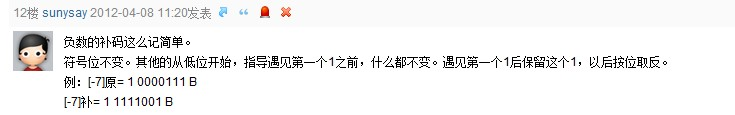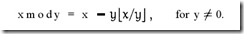# 二进制原码、反码、补码

## 简单小结

`65`为例（`8位`），其二进制形式为`01000001`（由于是8位所以在前面补了0）。

• 符号位指从左到右第一位，在这为`0`，也叫最高位，当然最低位就是从右至左第一位了。符号位为`1`时代表正数，为`0`时代表负数。
• 真值即指除了符号位后的二进制数，在这为`1000001`，也是我们平时所写的形式。

### 原码

`+1000001` = `01000001[原]` （+65）
`-1000001` = `11000001[原]` （-65）

【注意】：正数的原码、补码与反码均相同。

### 反码

`+1000001` = `01000001[反]` （+65）【同原码】
`-1000001` = `10111110[反]` （-65）

### 补码

`+1000001` = `01000001[补]` （+65）【同原码】
`-1000001` = `10111111[补]` （-65）

【负数补码小技巧】技巧确实好用，指导两字更正为直到。

【说明】：0的原码跟反码都有两个，因为这里0被分为+0和-0，0的补码是唯一的，8位时`0[补]`=`00000000`

### 补充：补码运算

`[X1+X2]补 = [x1]补 + [x2]补`
`[X1-X2]补 = [x1]补 + [-x2]补`

1. 补码运算时，其符号位与数值部分一起参加运算。
2. 补码的符号位相加后，如果有进位出现，要把这个进位舍去（自然丢失）。
3. 用补码运算，其运算结果亦为补码。在转换为真值时，若符号位为0，数位不变；若符号位为1，应将结果求补才是其真值。

`[X]补 = 01101``[-Y]补 = 11010`，则`[Z]补 = [X]补+[-Y]补 = 01101+11010 = 100111` , 其真值为`Z = + 0111`

`[X]补 = 00110``[-Y]补 = 10011`，则`[Z]补 =[X]补+[-Y]补 = 00110 + 10011 = 11001`, 其真值为`Z = - 0111`

【参考资料】：

【转载申明】

## 一. 机器数和真值

### 2、真值

`因为第一位是符号位，所以机器数的形式值就不等于真正的数值。例如上面的有符号数10000011，其最高位1代表负，其真正数值是 -3 而不是形式值131（10000011转换成十进制等于131）。所以，为区别起见，将带符号位的机器数对应的真正数值称为机器数的真值。`

## 二. 原码, 反码, 补码的基础概念和计算方法

### 1. 原码

`[+1]原 = 0000 0001`
`[-1]原 = 1000 0001`

### 2. 反码

`[+1] = 原 = 反`
`[-1] = 原 = 反`

### 3. 补码

`[+1] = 原 = 反 = 补`
`[-1] = 原 = 反 = 补`

## 三. 为何要使用原码, 反码和补码

`[+1] = 原 = 反 = 补`

`[-1] = 原 = 反 = 补`

`1 - 1 = 1 + (-1) = 原 + 原 = 原 = -2`

`1 - 1 = 1 + (-1) = [0000 0001]原 + [1000 0001]原= [0000 0001]反 + [1111 1110]反 = [1111 1111]反 = [1000 0000]原 = -0`

`1-1 = 1 + (-1) = [0000 0001]原 + [1000 0001]原 = [0000 0001]补 + [1111 1111]补 = [0000 0000]补=[0000 0000]原`

`(-1) + (-127) = [1000 0001]原 + [1111 1111]原 = [1111 1111]补 + [1000 0001]补 = [1000 0000]补`

-1-127的结果应该是-128, 在用补码运算的结果中, `[1000 0000]补` 就是-128. 但是注意因为实际上是使用以前的-0的补码来表示-128, 所以-128并没有原码和反码表示.(对-128的补码表示`[1000 0000]补`算出来的原码是`[0000 0000]原`, 这是不正确的)

## 四 原码, 反码, 补码 再深入

1. 往回拨2个小时: 6 – 2 = 4
2. 往前拨10个小时: (6 + 10) mod 12 = 4

3. 往前拨10+12=22个小时: (6+22) mod 12 =4

2,3方法中的mod是指取模操作, 16 mod 12 = 4 即用16除以12后的余数是4.

### 同余的概念

1. 4 mod 12 = 4
2. 16 mod 12 = 4

3. 28 mod 12 = 4

### 负数取模`x mod y = x - y L x / y J`

x mod y等于 x 减去 y 乘上 x与y的商的下界.

-3 mod 2

`= -3 - 2xL -3/2 J`

`= -3 - 2xL-1.5J`

`= -3 - 2x(-2)`

`= -3 + 4 = 1`

`(-2) mod 12 = 12-2=10`

`(-4) mod 12 = 12-4 = 8`

`(-5) mod 12 = 12 - 5 = 7`

### 开始证明

`回拨2小时 = 前拨10小时`

`回拨4小时 = 前拨8小时`

`回拨5小时= 前拨7小时`

(-2) mod 12 = 10

10 mod 12 = 10

-2与10是同余的.

`(-4) mod 12 = 8`

`8 mod 12 = 8`

-4与8是同余的.

`a ≡ a (mod m)`

(1)`a ± c ≡ b ± d (mod m)`

(2)`a * c ≡ b * d (mod m)`

`7 ≡ 7 (mod 12)`

`(-2) ≡ 10 (mod 12)`

`7 -2 ≡ 7 + 10 (mod 12)`

`2-1=2+(-1) = [0000 0010]原 + [1000 0001]原= [0000 0010]反 + [1111 1110]反`

`(-1) mod 127 = 126`

`126 mod 127 = 126`

`(-1) ≡ 126 (mod 127)`

`2-1 ≡ 2+126 (mod 127)`

2-1 与 2+126的余数结果是相同的! 而这个余数, 正式我们的期望的计算结果: 2-1=1

`2-1=2+(-1) = [0000 0010]原 + [1000 0001]原 = [0000 0010]补 + [1111 1111]补`

`[0111 1111]原 = 127`

`(-1) mod 128 = 127`

`127 mod 128 = 127`

`2-1 ≡ 2+127 (mod 128)`

The end.
2017-09-15 星期五

Not Comment Found